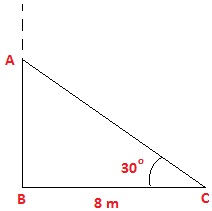Guru

# A tree breaks due to storm and the broken part bends so that the top of the tree touches the ground making an angle 30° with it. The distance between the foot of the tree to the point where the top touches the ground is 8 m. Find the height of the tree. Q.2

• 1

Sir please help me to solve the problem of the applications of trigonometry chapter of class 10th ncert math of exercise 9.1 of question no.2 give me the best way to solve this question A tree breaks due to storm and the broken part bends so that the top of the tree touches the ground making an angle 30° with it. The distance between the foot of the tree to the point where the top touches the ground is 8 m. Find the height of the tree.

Share

1. Using given instructions, draw a figure. Let AC be the broken part of the tree. Angle C = 30°

BC = 8 m

To Find: Height of the tree, which is ABFrom figure: Total height of the tree is the sum of AB and AC i.e. AB+AC

In right ΔABC,

Using Cosine and tangent angles,

cos 30° = BC/AC

We know that, cos 30° = √3/2

√3/2 = 8/AC

AC = 16/√3 …(1)

Also,

tan 30° = AB/BC

1/√3 = AB/8

AB = 8/√3 ….(2)

Therefore, total height of the tree = AB + AC = 16/√3 + 8/√3 = 24/√3 = 8√3 m.

• 1